• 假如某个观察值序列通过序列预处理可以判定为平稳白噪声序列，就可以利用ARMA模型对该序列进行建模建模的基本步骤如下：（1）求出该观察值序列的样本自相关系数（ACF）和样本偏自相关系数（PACF）的值。（2）...
假如某个观察值序列通过序列预处理可以判定为平稳非白噪声序列，就可以利用ARMA模型对该序列进行建模。建模的基本步骤如下：（1）求出该观察值序列的样本自相关系数（ACF）和样本偏自相关系数（PACF）的值。（2）根据样本自相关系数和偏自相关系数的性质，选择适当的ARMA（p，q）模型进行拟合。（3）估计模型中位置参数的值。（4）检验模型的有效性。如果模型不通过检验，转向步骤（2），重新选择模型再拟合。（5）模型优化。如果拟合模型通过检验，仍然转向不走（2），充分考虑各种情况，建立多个拟合模型，从所有通过检验的拟合模型中选择最优模型。（6）利用拟合模型，预测序列的将来走势。二、代码实现1、绘制时序图，查看数据的大概分布trainSeting.head()Out:date2017-10-01 126.42017-10-02 82.42017-10-03 78.12017-10-04 51.12017-10-05 90.9Name: sales, dtype: float64plt.plot(trainSeting)2、平稳性检验'''进行ADF检验adf_test的返回值Test statistic：代表检验统计量p-value：代表p值检验的概率Lags used：使用的滞后k，autolag=AIC时会自动选择滞后Number of Observations Used：样本数量Critical Value(5%) : 显著性水平为5%的临界值。(1)假设是存在单位根，即不平稳；(2)显著性水平，1%：严格拒绝原假设；5%：拒绝原假设，10%类推。(3)看P值和显著性水平a的大小，p值越小，小于显著性水平的话，就拒绝原假设，认为序列是平稳的；大于的话，不能拒绝，认为是不平稳的(4)看检验统计量和临界值，检验统计量小于临界值的话，就拒绝原假设，认为序列是平稳的；大于的话，不能拒绝，认为是不平稳的'''#滚动统计def rolling_statistics(timeseries):#Determing rolling statisticsrolmean = pd.rolling_mean(timeseries, window=12)rolstd = pd.rolling_std(timeseries, window=12)#Plot rolling statistics:orig = plt.plot(timeseries, color='blue',label='Original')mean = plt.plot(rolmean, color='red', label='Rolling Mean')std = plt.plot(rolstd, color='black', label = 'Rolling Std')plt.legend(loc='best')plt.title('Rolling Mean & Standard Deviation')plt.show(block=False)##ADF检验from statsmodels.tsa.stattools import adfullerdef adf_test(timeseries):rolling_statistics(timeseries)#绘图print ('Results of Augment Dickey-Fuller Test:')dftest = adfuller(timeseries, autolag='AIC')dfoutput = pd.Series(dftest[0:4], index=['Test Statistic','p-value','#Lags Used','Number of Observations Used'])for key,value in dftest.items():dfoutput['Critical Value (%s)'%key] = value #增加后面的显著性水平的临界值print (dfoutput)adf_test(trainSeting) #从结果中可以看到p值为0.1097>0.1，不能拒绝H0,认为该序列不是平稳序列返回结果如下Results of Augment Dickey-Fuller Test:Test Statistic? ? -5.718539e+00p-value? ? ? 7.028398e-07#Lags Used? ? ? 0.000000e+00Number of Observations Used 6.200000e+01Critical Value (1%)? -3.540523e+00Critical Value (5%)? -2.909427e+00Critical Value (10%)? -2.592314e+00dtype: float64通过上面可以看到，p值小于0.05，可以认为该序列为平稳时间序列。3、白噪声检验'''acorr_ljungbox(x, lags=None, boxpierce=False)函数检验无自相关lags为延迟期数，如果为整数，则是包含在内的延迟期数，如果是一个列表或数组，那么所有时滞都包含在列表中最大的时滞中boxpierce为True时表示除开返回LB统计量还会返回Box和Pierce的Q统计量返回值：lbvalue:测试的统计量pvalue:基于卡方分布的p统计量bpvalue:((optionsal), float or array) – test statistic for Box-Pierce testbppvalue:((optional), float or array) – p-value based for Box-Pierce test on chi-square distribution'''from statsmodels.stats.diagnostic import acorr_ljungboxdef test_stochastic(ts,lag):p_value = acorr_ljungbox(ts, lags=lag) #lags可自定义return p_valuetest_stochastic(trainSeting,[6,12])Out: (array([13.28395274, 14.89281684]), array([0.03874194, 0.24735042]))从上面的分析结果中可以看到，延迟6阶的p值为0.03<0.05，因此可以拒绝原假设，认为该序列不是白噪声序列。4、确定ARMA的阶数（1）利用自相关图和偏自相关图####自相关图ACF和偏相关图PACFimport statsmodels.api as smdef acf_pacf_plot(ts_log_diff):sm.graphics.tsa.plot_acf(ts_log_diff,lags=40) #ARIMA,qsm.graphics.tsa.plot_pacf(ts_log_diff,lags=40) #ARIMA,pacf_pacf_plot(trainSeting) #查看数据的自相关图和偏自相关图（2）借助AIC、BIC统计量自动确定##借助AIC、BIC统计量自动确定from statsmodels.tsa.arima_model import ARMAdef proper_model(data_ts, maxLag):init_bic = float("inf")init_p = 0init_q = 0init_properModel = Nonefor p in np.arange(maxLag):for q in np.arange(maxLag):model = ARMA(data_ts, order=(p, q))try:results_ARMA = model.fit(disp=-1, method='css')except:continuebic = results_ARMA.bicif bic < init_bic:init_p = pinit_q = qinit_properModel = results_ARMAinit_bic = bicreturn init_bic, init_p, init_q, init_properModelproper_model(trainSeting,40)#在statsmodels包里还有更直接的函数：import statsmodels.tsa.stattools as storder = st.arma_order_select_ic(ts_log_diff2,max_ar=5,max_ma=5,ic=['aic', 'bic', 'hqic'])order.bic_min_order'''我们常用的是AIC准则，AIC鼓励数据拟合的优良性但是尽量避免出现过度拟合(Overfitting)的情况。所以优先考虑的模型应是AIC值最小的那一个模型。为了控制计算量，我们限制AR最大阶不超过5，MA最大阶不超过5。 但是这样带来的坏处是可能为局部最优。timeseries是待输入的时间序列，是pandas.Series类型，max_ar、max_ma是p、q值的最大备选值。order.bic_min_order返回以BIC准则确定的阶数，是一个tuple类型返回值如下：order.bic_min_orderOut: (1, 0)5、建模从上述结果中可以看到，可以选择AR(1)模型################################模型####################################### AR模型，q=0#RSS是残差平方和# disp为-1代表不输出收敛过程的信息，True代表输出from statsmodels.tsa.arima_model import ARIMAmodel = ARIMA(trainSeting,order=(1,0,0)) #第二个参数代表使用了二阶差分results_AR = model.fit(disp=-1)plt.plot(trainSeting)plt.plot(results_AR.fittedvalues, color='red') #红色线代表预测值plt.title('RSS:%.4f' % sum((results_AR.fittedvalues-trainSeting)**2))#残差平方和6、预测未来走势############################预测未来走势########################################### forecast方法会自动进行差分还原，当然仅限于支持的1阶和2阶差分forecast_n = 12 #预测未来12个天走势forecast_AR = results_AR.forecast(forecast_n)forecast_AR = forecast_ARprint (forecast_AR)print (forecast_ARIMA_log)[90.49452199 84.05407353 81.92752342 81.22536496 80.99352161 80.9169700380.89169372 80.88334782 80.88059211 80.87968222 80.87938178 80.87928258]##将预测的数据和原来的数据绘制在一起，为了实现这一目的，我们需要增加数据索引，使用开源库arrow:import arrowdef get_date_range(start, limit, level='day',format='YYYY-MM-DD'):start = arrow.get(start, format)result=(list(map(lambda dt: dt.format(format) , arrow.Arrow.range(level, start,limit=limit))))dateparse2 = lambda dates:pd.datetime.strptime(dates,'%Y-%m-%d')return map(dateparse2, result)# 预测从2017-12-03开始，也就是我们训练数据最后一个数据的后一个日期new_index = get_date_range('2017-12-03', forecast_n)forecast_ARIMA_log = pd.Series(forecast_AR, copy=True, index=new_index)print (forecast_ARIMA_log.head())##绘图如下plt.plot(trainSeting,label='Original',color='blue')plt.plot(forecast_ARIMA_log, label='Forcast',color='red')plt.legend(loc='best')plt.title('forecast')以上这篇利用python实现平稳时间序列的建模方式就是小编分享给大家的全部内容了，希望能给大家一个参考，也希望大家多多支持。
展开全文• 假如某个观察值序列通过序列预处理可以判定为平稳白噪声序列，就可以利用ARMA模型对该序列进行建模建模的基本步骤如下： （1）求出该观察值序列的样本自相关系数（ACF）和样本偏自相关系数（PACF）的值。 （2）...
• 对于平稳多元时间序列建模的思想是：假定响应序列{yt}和输入序列{x1t},{x2t},…,{xkt}均平稳，首先构造响应序列和输入序列的回归模型： 因为响应序列和输入序列皆是平稳序列，那么其残差序列也是平稳序列： 上述...
1.模型简介
对于平稳多元时间序列建模的思想是：假定响应序列{yt}和输入序列{x1t},{x2t},…,{xkt}均平稳，首先构造响应序列和输入序列的回归模型：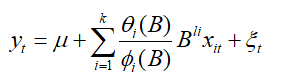因为响应序列和输入序列皆是平稳序列，那么其残差序列也是平稳序列：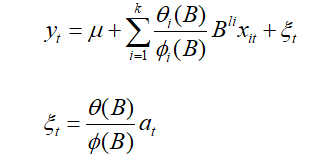上述模型称为动态回归模型，简记ARIMAX.
2.建模步骤
（1）读入数据，并绘制其时序图，并判断其平稳性
（2）绘制其输出序列自相关图，偏自相关图，并利用其自相关图和偏自相关图给模型定阶。
（3）对输出序列拟合模型
（4)  对输出序列进行残差白噪声检验
（5）画出输入序列和输出序列的协相关图
（6)如果输入序列和输出序列具有长期的协相关关系，拟合输入序列和输出序列的线性模型。
3.建模
我们以天然气输入速率为输入序列，建立CO2的输出百分浓度模型。
（1）画出其时序图，判断其平稳性。
library(tseries)
library(zoo)
library(forecast)
a
y=ts(a$锘縪utput) plot(y,col=4,lwd=2,pch=8,type="o") adf.test(y)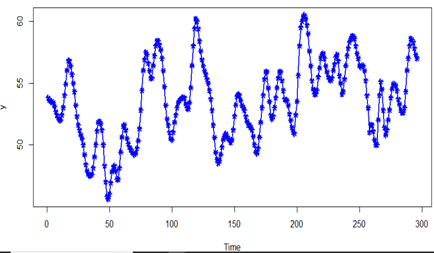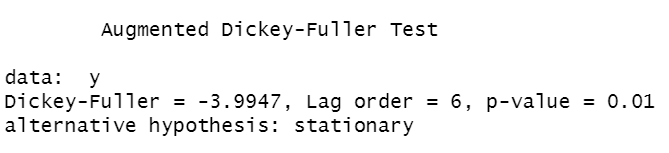检验结果可知该序列为平稳序列。 （2)画出其自相关图和偏自相关图 acf(y,col=4,lwd=2) pacf(y,col=4,lwd=2)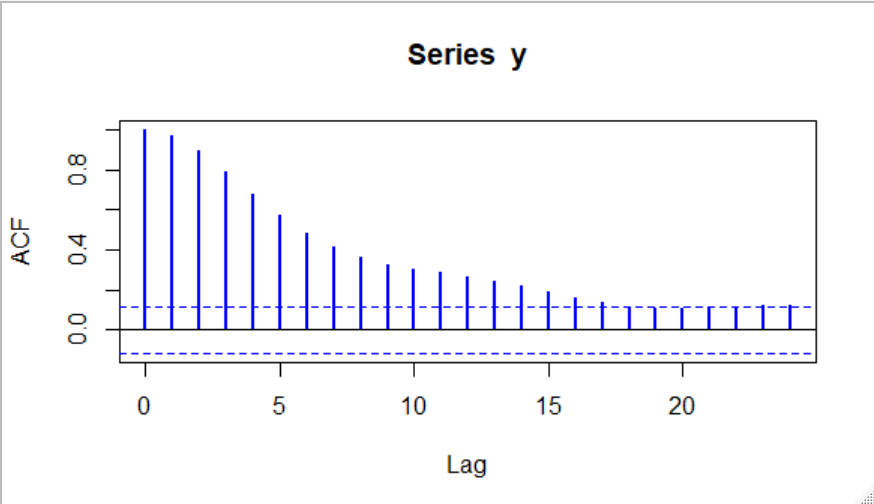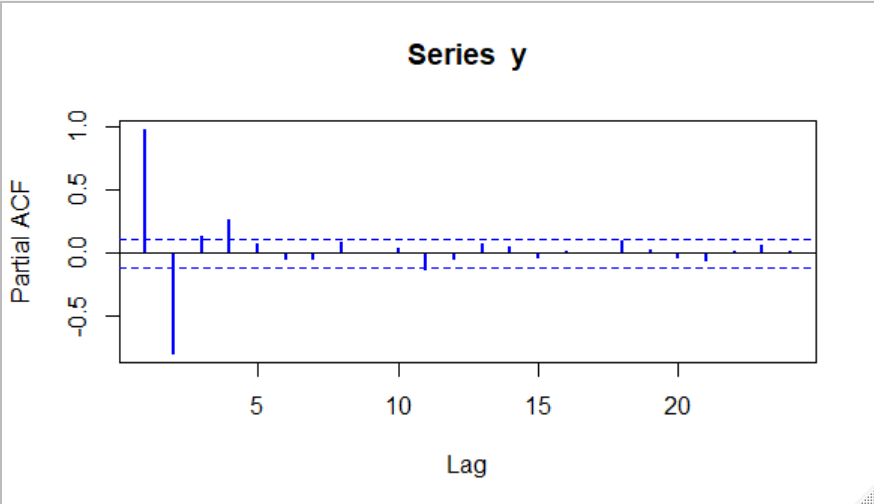可以看出自相关图拖尾，偏自相关图4阶截尾，所以对输出序列Y 拟合AR(4)模型。 （3)对输出序列拟合AR(4)模型 y.fit=arima(y,order=c(4,0,0)) y.fit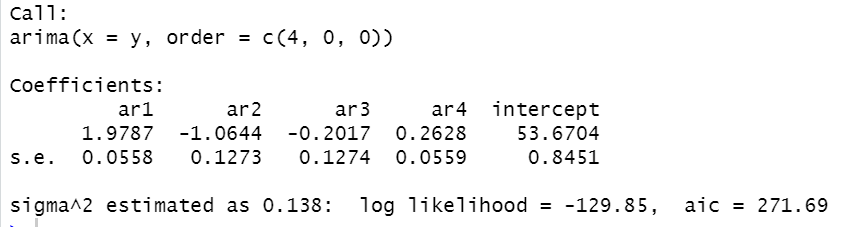对参数进行显著性检验： t1=1.9787/0.0558 pt(t1,df=293,lower.tail=F) t2=-1.0644/0.1273 pt(t2,df=293,lower.tail=T) t3=-0.2017/0.1274 pt(t3,df=293,lower.tail=T) t4=0.2628/0.0559 pt(t4,df=293,lower.tail=F)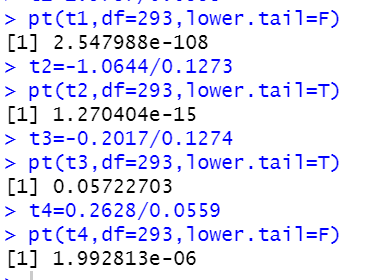参数检验基本通过 对残差进行白噪声检验： for(i in 1:3) print(Box.test(y.fit$residual,lag=6*i))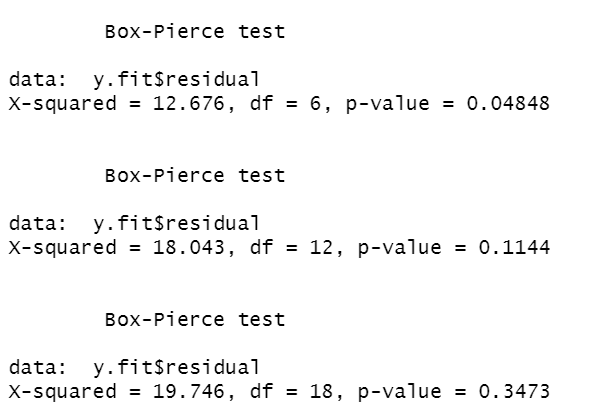（4）画出输入序列和输出序列的协相关图。
x=ts(a$input) ccf(y,x,col=4,lwd=2)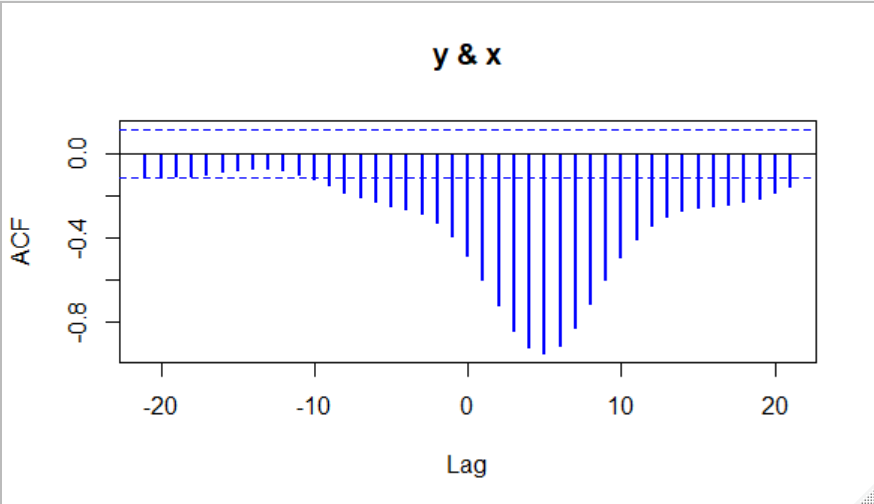ccf函数中x序列和y序列的位置可以随意放置，协相关图的形状不变。ccf（x,y)表示x比y 的滞后情况，ccf(y,x)表示y比x的滞后情况。上图显示y比x滞后5期左右。 可以看出，输入序列和输出序列有着长期的协相关关系。 （5）拟合模型： 本例中，对输入序列采用AR(2,1)的转移函数结构，残差部分依然是AR(4)模型，拟合结果如下： y.fit2=arimax(y,order=c(4,0,0),xtrans=x,transfer=list(c(2,1))) y.fit2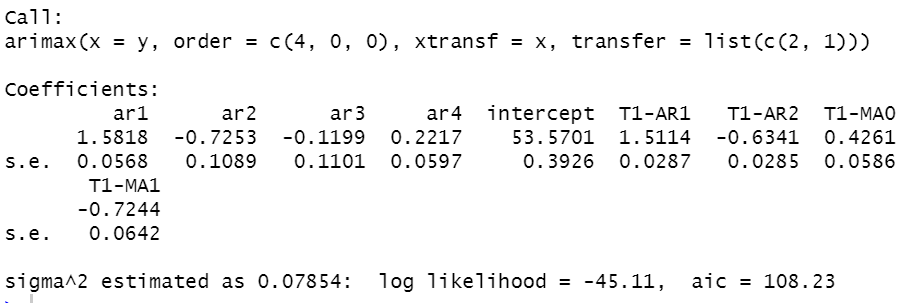拟合的模型为：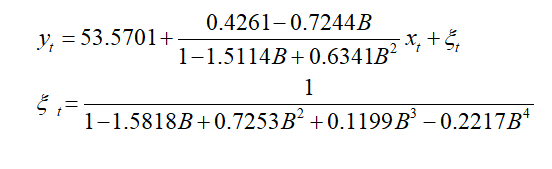对回归残差序列进行白噪声检验： for(i in 1:3) print(Box.test(y.fit2$residual,lag=6*i))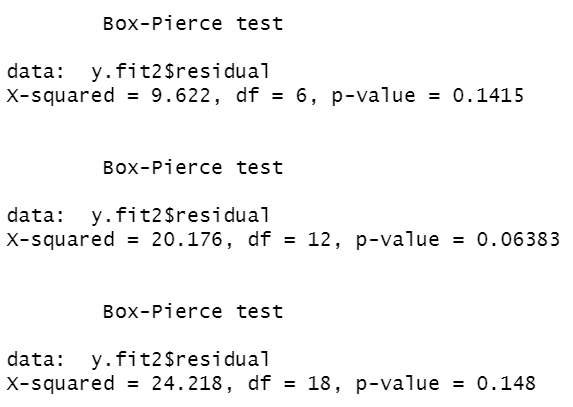展开全文•  假如某个观察值序列通过序列预处理可以判定为平稳白噪声序列，就可以利用ARMA模型对该序列进行建模建模的基本步骤如下： （1）求出该观察值序列的样本自相关系数（ACF）和样本偏自相关系数（PACF）的值。 ...
一、平稳序列建模步骤

假如某个观察值序列通过序列预处理可以判定为平稳非白噪声序列，就可以利用ARMA模型对该序列进行建模。建模的基本步骤如下：

（1）求出该观察值序列的样本自相关系数（ACF）和样本偏自相关系数（PACF）的值。

（2）根据样本自相关系数和偏自相关系数的性质，选择适当的ARMA（p，q）模型进行拟合。

（3）估计模型中位置参数的值。

（4）检验模型的有效性。如果模型不通过检验，转向步骤（2），重新选择模型再拟合。

（5）模型优化。如果拟合模型通过检验，仍然转向不走（2），充分考虑各种情况，建立多个拟合模型，从所有通过检验的拟合模型中选择最优模型。

（6）利用拟合模型，预测序列的将来走势。

二、代码实现

1、绘制时序图，查看数据的大概分布

Out:
date
2017-10-01    126.4
2017-10-02     82.4
2017-10-03     78.1
2017-10-04     51.1
2017-10-05     90.9
Name: sales, dtype: float64

plt.plot(trainSeting)

￼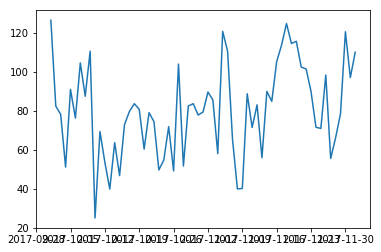2、平稳性检验

'''进行ADF检验
Test statistic：代表检验统计量
p-value：代表p值检验的概率
Lags used：使用的滞后k，autolag=AIC时会自动选择滞后
Number of Observations Used：样本数量
Critical Value(5%) : 显著性水平为5%的临界值。

(1)假设是存在单位根，即不平稳；
(2)显著性水平，1%：严格拒绝原假设；5%：拒绝原假设，10%类推。
(3)看P值和显著性水平a的大小，p值越小，小于显著性水平的话，就拒绝原假设，认为序列是平稳的；大于的话，不能拒绝，认为是不平稳的
(4)看检验统计量和临界值，检验统计量小于临界值的话，就拒绝原假设，认为序列是平稳的；大于的话，不能拒绝，认为是不平稳的
'''
#滚动统计
def rolling_statistics(timeseries):
#Determing rolling statistics
rolmean = pd.rolling_mean(timeseries, window=12)
rolstd = pd.rolling_std(timeseries, window=12)

#Plot rolling statistics:
orig = plt.plot(timeseries, color='blue',label='Original')
mean = plt.plot(rolmean, color='red', label='Rolling Mean')
std = plt.plot(rolstd, color='black', label = 'Rolling Std')
plt.legend(loc='best')
plt.title('Rolling Mean & Standard Deviation')
plt.show(block=False)

rolling_statistics(timeseries)#绘图
print ('Results of Augment Dickey-Fuller Test:')
dfoutput = pd.Series(dftest[0:4], index=['Test Statistic','p-value','#Lags Used','Number of Observations Used'])
for key,value in dftest.items():
dfoutput['Critical Value (%s)'%key] = value   #增加后面的显著性水平的临界值
print (dfoutput)

adf_test(trainSeting)   #从结果中可以看到p值为0.1097>0.1，不能拒绝H0,认为该序列不是平稳序列

返回结果如下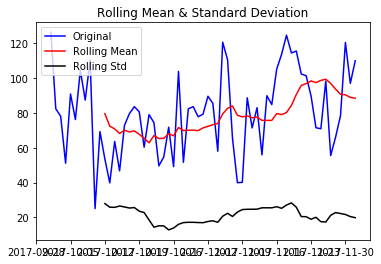Results of Augment Dickey-Fuller Test:
Test Statistic                -5.718539e+00
p-value                        7.028398e-07
#Lags Used                     0.000000e+00
Number of Observations Used    6.200000e+01
Critical Value (1%)           -3.540523e+00
Critical Value (5%)           -2.909427e+00
Critical Value (10%)          -2.592314e+00
dtype: float64

通过上面可以看到，p值小于0.05，可以认为该序列为平稳时间序列。

3、白噪声检验

'''acorr_ljungbox(x, lags=None, boxpierce=False)函数检验无自相关
lags为延迟期数，如果为整数，则是包含在内的延迟期数，如果是一个列表或数组，那么所有时滞都包含在列表中最大的时滞中
boxpierce为True时表示除开返回LB统计量还会返回Box和Pierce的Q统计量
返回值：
lbvalue:测试的统计量
pvalue:基于卡方分布的p统计量
bpvalue:((optionsal), float or array) – test statistic for Box-Pierce test
bppvalue:((optional), float or array) – p-value based for Box-Pierce test on chi-square distribution
'''
from statsmodels.stats.diagnostic import acorr_ljungbox
def test_stochastic(ts,lag):
p_value = acorr_ljungbox(ts, lags=lag) #lags可自定义
return p_value

test_stochastic(trainSeting,[6,12])
Out: (array([13.28395274, 14.89281684]), array([0.03874194, 0.24735042]))

从上面的分析结果中可以看到，延迟6阶的p值为0.03<0.05，因此可以拒绝原假设，认为该序列不是白噪声序列。

4、确定ARMA的阶数

（1）利用自相关图和偏自相关图

####自相关图ACF和偏相关图PACF
import statsmodels.api as sm
def acf_pacf_plot(ts_log_diff):
sm.graphics.tsa.plot_acf(ts_log_diff,lags=40) #ARIMA,q
sm.graphics.tsa.plot_pacf(ts_log_diff,lags=40) #ARIMA,p

acf_pacf_plot(trainSeting)   #查看数据的自相关图和偏自相关图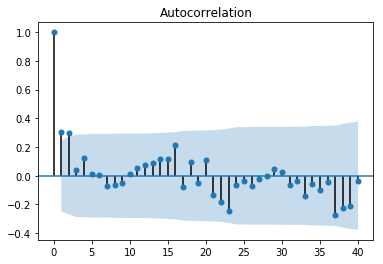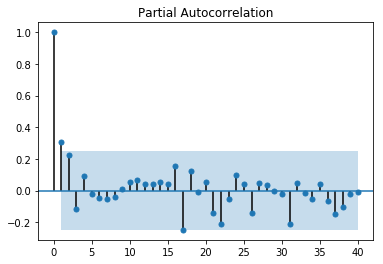（2）借助AIC、BIC统计量自动确定

##借助AIC、BIC统计量自动确定
from statsmodels.tsa.arima_model import ARMA
def proper_model(data_ts, maxLag):
init_bic = float("inf")
init_p = 0
init_q = 0
init_properModel = None
for p in np.arange(maxLag):
for q in np.arange(maxLag):
model = ARMA(data_ts, order=(p, q))
try:
results_ARMA = model.fit(disp=-1, method='css')
except:
continue
bic = results_ARMA.bic
if bic < init_bic:
init_p = p
init_q = q
init_properModel = results_ARMA
init_bic = bic
return init_bic, init_p, init_q, init_properModel

proper_model(trainSeting,40)

#在statsmodels包里还有更直接的函数：
import statsmodels.tsa.stattools as st
order = st.arma_order_select_ic(ts_log_diff2,max_ar=5,max_ma=5,ic=['aic', 'bic', 'hqic'])
order.bic_min_order
'''
我们常用的是AIC准则，AIC鼓励数据拟合的优良性但是尽量避免出现过度拟合(Overfitting)的情况。所以优先考虑的模型应是AIC值最小的那一个模型。
为了控制计算量，我们限制AR最大阶不超过5，MA最大阶不超过5。 但是这样带来的坏处是可能为局部最优。
timeseries是待输入的时间序列，是pandas.Series类型，max_ar、max_ma是p、q值的最大备选值。
order.bic_min_order返回以BIC准则确定的阶数，是一个tuple类型

返回值如下：

order.bic_min_order
Out: (1, 0)

5、建模

从上述结果中可以看到，可以选择AR(1)模型

################################模型######################################
# AR模型，q=0
# disp为-1代表不输出收敛过程的信息，True代表输出
from statsmodels.tsa.arima_model import ARIMA
model = ARIMA(trainSeting,order=(1,0,0)) #第二个参数代表使用了二阶差分
results_AR = model.fit(disp=-1)
plt.plot(trainSeting)
plt.plot(results_AR.fittedvalues, color='red') #红色线代表预测值
plt.title('RSS:%.4f' % sum((results_AR.fittedvalues-trainSeting)**2))#残差平方和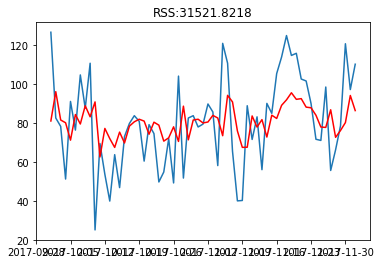6、预测未来走势

############################预测未来走势##########################################
# forecast方法会自动进行差分还原，当然仅限于支持的1阶和2阶差分
forecast_n = 12 #预测未来12个天走势
forecast_AR = results_AR.forecast(forecast_n)
forecast_AR = forecast_AR
print (forecast_AR)

print (forecast_ARIMA_log)
[90.49452199 84.05407353 81.92752342 81.22536496 80.99352161 80.91697003

80.89169372 80.88334782 80.88059211 80.87968222 80.87938178 80.87928258]

##将预测的数据和原来的数据绘制在一起，为了实现这一目的，我们需要增加数据索引，使用开源库arrow:
import arrow
def get_date_range(start, limit, level='day',format='YYYY-MM-DD'):
start = arrow.get(start, format)
result=(list(map(lambda dt: dt.format(format) , arrow.Arrow.range(level, start,limit=limit))))
dateparse2 = lambda dates:pd.datetime.strptime(dates,'%Y-%m-%d')
return map(dateparse2, result)

# 预测从2017-12-03开始，也就是我们训练数据最后一个数据的后一个日期
new_index = get_date_range('2017-12-03', forecast_n)
forecast_ARIMA_log = pd.Series(forecast_AR, copy=True, index=new_index)
##绘图如下
plt.plot(trainSeting,label='Original',color='blue')
plt.plot(forecast_ARIMA_log, label='Forcast',color='red')
plt.legend(loc='best')
plt.title('forecast')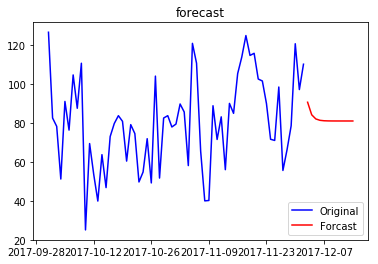展开全文python
• 平稳时间序列建模1.建模步骤2.根据序列的时序图，自相关图和偏自相关图判断序列的平稳性，随机性2.1.时序图检验2.2.自相关图检验2.3判断该序列的纯随机性3.选择适当模型4.参数估计5.模型检验及优化5.1模型显著性...
时间序列分析：平稳时间序列分析一.录入数据1.读入数据2.转为时间序列数据二. 平稳时间序列建模1.建模步骤2.根据序列的时序图，自相关图和偏自相关图判断序列的平稳性，随机性2.1.时序图检验2.2.自相关图检验2.3判断该序列的纯随机性3.选择适当模型4.模型检验及优化4.1模型显著性检验4.2参数显著性检验4.3模型优化三.时间序列预测
一.录入数据
1.读入数据
在R中输入一下命令:
rain=read.table("/home/ddy/桌面/习题3.17数据.txt",sep="\t",header = F)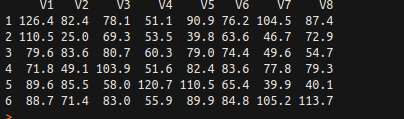我们发现该数据录入的时候是按行录入的，我们需要的时按列录入的数据，所以我们对原数据先转置，再将数据的每一列链接到第一列下面，代码实现如下：
#将数据转置
> rain=t(rain)
> rain_m=matrix(data=0, nrow = 64, ncol = 1)
> k=1
#将数据的每一列链接到第一列下面
> for(i in 1:8){
for(j in 1:8){
rain_m[k]=rain[j,i]
k=k+1
}
}
> rain_m

2.转为时间序列数据
rain_m=ts(rain_m)

二. 平稳时间序列建模
1.建模步骤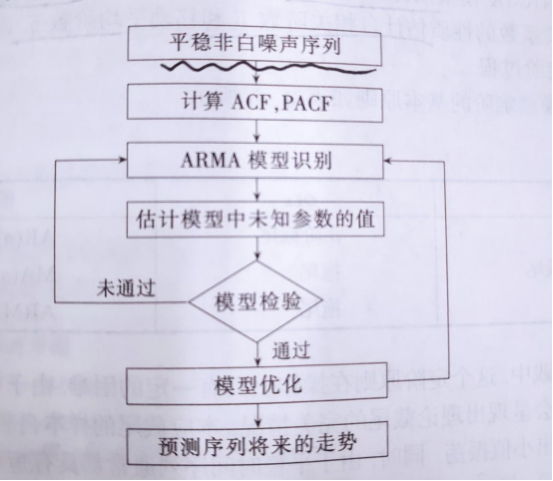2.根据序列的时序图，自相关图和偏自相关图判断序列的平稳性，随机性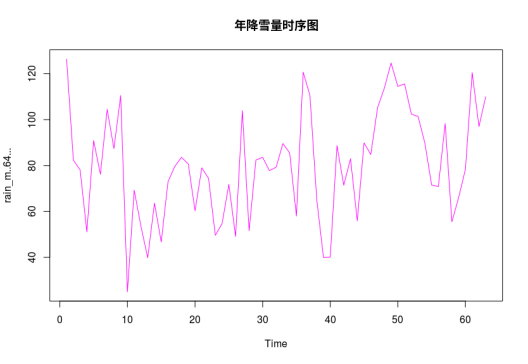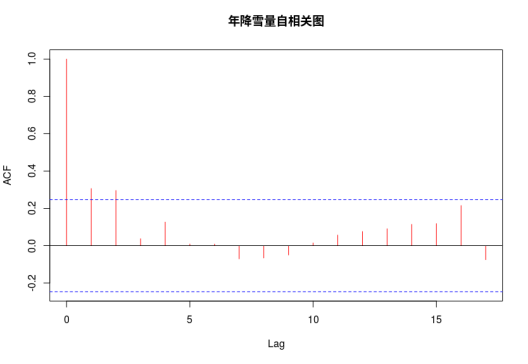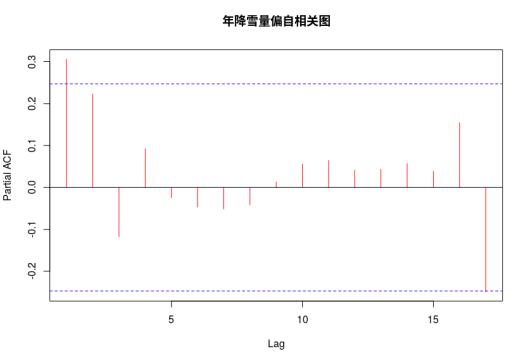2.1.时序图检验
由时序图可初步得知该地区年降雨量几乎都在80在右波动，没有明显的周期性和单调性，所以认为该序列为平稳序列。
2.2.自相关图检验
在自相关图中，我们发现除了延迟1,2阶的自相关系数在2倍标准差以外，其他阶数的自相关系数2倍标准差以内波动，根据自相关系数的这个特点可以判断该序列具有短期相关性，即认为该序列为平稳序列。
并且非0自相关系数衰减到小值是一个突然的过程，不是一个连续渐变的过程，这是自相关系数截尾的的典型特征。
在偏自相关系数衰减到0的过程比较缓慢，可知道偏自相关系数不截尾。
综合1,2我们可认为该地区年降雨量序列是平稳序列。
2.3判断该序列的纯随机性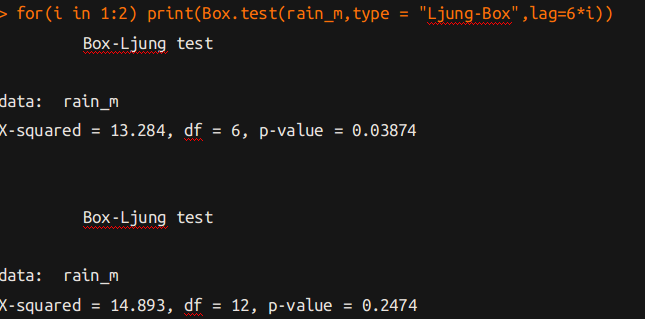根据检验结果我们可以得出，从延迟6阶的检验结果中我们可以把得到P值都小于显著性水平0.05。因此，我们拒绝序列纯随机的原假设，即该序列为非白噪声序列，我们可以认为该该地区年降雪量序列变动不属于随机变动，这说明我们可以根据历史信息来预测未来年份的该地区年降雪量。
3.选择适当模型
在自相关系数衰减到0的过程，除了延迟2阶的偏自相关系数在2倍标准差以外，其他阶数的偏自相关系数2倍标准差以内波动，这是一个自相关系数2阶拖尾的的典型特征。
所以我们选择初步用MA(2) 模型来拟合序列，为了尽量避免因个人经验不足而导致模型识别不准确，所以我们运用auto.arima()函数来自动识别模型阶数，并从中得到参数估计值。
auto.arima(rain_m)的结果为：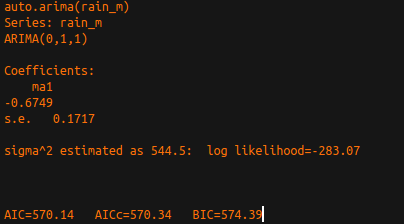由此可知，我们不能选择MA(2)模型，而要选择MA(1)模型，接下来我们对MA(1)模型进行检验：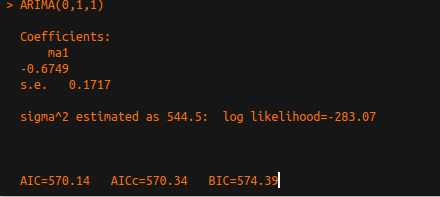4.模型检验及优化
4.1模型显著性检验
模型显著性检验只要是检验模型的有效性，一个模型是否显著有效主要看它提取的信息是否充分，一个好的拟合模型应该能够提取观察值序列中几乎所有的样本相关信息。换一句话说就是拟合残差项中将不在蕴含任何相关信息，即残差序列应该为白噪声序列，这样的模型才称为显著有效模型。所以我们可以认为模型的显著性检验就是残差序列的白噪声简检验。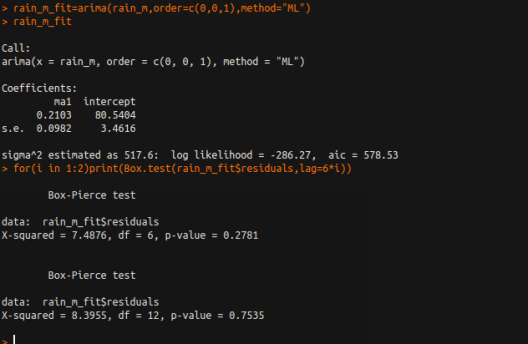由检验结果可知，各阶延迟下的LB统计量的P值都大于0.05,可以认为这个拟合模型的残差序列属于白噪声序列，即该拟合模型显著有效。
此外，即使通过了MA(1)模型的检验。为了充分考虑各种可能，我们建立多个拟合模型，我们发现MA(2)模型的结果并没有 MA(1)的结果显著，所以我们最终选择MA(1)模型进行拟合。
4.2参数显著性检验
检验函数：
pt(t,df = ,lower.tail = )

t:t统计量的值图（该例子中：t=0.2103/0.0982）
df:自由度（n-参数个数）
lower.tail:确定计算概率的方向。对于参数显著性检验，如果参数估计值为负，选择lower.tail=T

4.3模型优化
若一个模型通过里检验，说明在一定置信水平下，该模型能够有效的拟合观察值序列的波动，但这种模型并不是唯一的。此时我们可以通过比较各个模型的AIC值和SBC值来进行最优模型的选择。
三.时间序列预测
利用拟合模型，预测城市未来5年的降雪量：
> rain.fore=forecast(rain_m_fit,h=5)> plot(rain.fore)

得到该城市未来5年的降雪量预测图：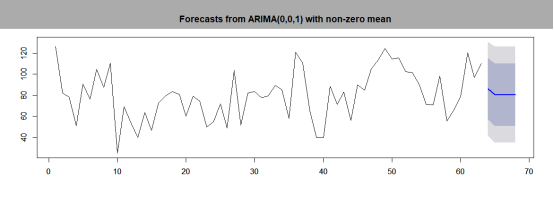代码汇总：

rain=t(rain)

rain_m=matrix(data=0, nrow = 64, ncol = 1)
k=1
for(i in 1:8){
for(j in 1:8){
rain_m[k]=rain[j,i]
k=k+1
}
}

rain_m=data.frame(rain_m[-64,])

rain_m=ts(rain_m)
par(mfcol=c(3,1))
plot(rain_m,main = "年降雪量时序图",col=6)
acf(rain_m,main = "年降雪量自相关图",col=2)
pacf(rain_m,main = "年降雪量偏自相关图",col=2)

#系统自动定阶
auto.arima(rain_m)

arima(x = rain_m, order = c(0, 0, 1), method = "ML")
for(i in 1:2)print(Box.test(rain_m_fit$residuals,lag=6*i)) pt( 0.2103/0.0982,df=62,lower.tail=F) rain.fore=forecast(rain_m_fit,h=5) rain.fore plot(rain.fore) ############ #预测图个性化# ############ L1<-x.fore$fitted-1.96*sqrt(x.fit$sigma2) U1<-x.fore$fitted+1.96*sqrt(x.fit$sigma2) L2<-ts(x.fore$lower[,2],start = 2009)
U2<-ts(x.fore$upper[,2],start = 2009) c1<-min(x,L1,L2) c2<-max(x,L2,U2) plot(x,type = "p",pch=8,xlim = c(1950,2013),ylim = c(c1,c2)) lines(x.fore$fitted,col=2,lwd=2)
lines(x.fore\$mean,col=2,lwd=2)
lines(L1,col=4,lty=2)
lines(L2,col=4,lty=2)
lines(U1,col=4,lty=2)
lines(U2,col=4,lty=2)




展开全文• 一、平稳序列建模步骤假如某个观察值序列通过序列预处理可以判定为平稳白噪声序列，就可以利用ARMA模型对该序列进行建模。建模的基本步骤如下：（1）求出该观察值序列的样本自相关系数（ACF）和样本偏自相关系数...
• 时间序列简而言之，时间序列就是带时间戳的数值序列。股票，期货等金融数据就是典型的时间序列。量化的过程，很多时间都是在分析时间序列，找到...平稳性是当前时间序列分析的前提条件，因为我们的建模过程基本都...
• 假如某个观察值序列通过序列预处理可以判定为平稳白噪声序列，就可以利用ARMA模型对该序列进行建模建模的基本步骤如下：（1）求出该观察值序列的样本自相关系数（ACF）和样本偏自相关系数（PACF）的值。（2）...
• 时间序列简而言之，时间序列就是带时间戳的数值序列。股票，期货等金融数据就是典型的时间序列。量化的过程，很多时间都是在分析时间序列，找到...平稳性是当前时间序列分析的前提条件，因为我们的建模过程基本都...
• [时间序列分析]--平稳性,白噪声的检验  这是一个全新的专题，讲关于时间序列分析的。还是老规矩，我使用mathematica来实现。    我个人认为时间序列分析是一门挺重要的科目，如果做建模什么的一定是知道的，...
•  残差白噪声检验，模型系数显著性检验  用验证集数据来验证 模型预测  模型确定，用于预测   案例 > plot(tsdata) #做时序图观察，是否为平稳序列，其实可以直接adf.test() 检测，如果显著...
• 时间序列 简而言之，时间序列就是带时间戳的数值序列。...所谓时间序列平稳性，是指时间序列的均值，方差以及协方差都是常数，与时间t无关。这样的序列才可以作为我们基于历史预测未来的基础。 满足以上条件属...
• ARMA 模型Auto-Regressive and Moving Average Model是研究时间序列的重要方法由自回归模型简称AR模型与滑动平均模型简称MA模型为基础“混合”构成。在市场研究中常用于长期追踪资料的研究如Panel研究中用于消费行为...python 数据库
• 【参考资料】 【1】《应用时序分析》 【2】https://pandas.pydata.org/pandas-docs/stable/api.html 【3】测试数据来源:智能运维挑战赛 http://iops.ai/ ...当序列经过预处理被认为是一个平稳白噪声的时...人工智能 ARMA python
• 白噪声检验也称为纯随机性检验， 当数据是纯随机数据时，再对数据进行分析就没有任何意义了， 所以拿到数据后最好对数据进行一个纯随机性检验acorr_ljungbox(x, lags=None, boxpierce=False) # 数据的纯随机性检验...
• 平稳序列建模 零、基本概念 1.两种方法工具 →→{\to}差分运算 →→{\to}延迟算子 ...考察非白噪声序列→→{\to}根据该序列自相关图以及偏自相关系数图→→{\to}选择合适ARMA模型...
• 时间序列建模流程时间序列的建模分析流程时间序列可视化序列平稳平稳平稳的区别差分法处理非平稳数据模型自回归模型（AR）移动平均模型（MA）自回归平均模型（ARMA）差分自回归移动平均模型（ARIMA）通过ACF/...机器学习
• 白噪声检验也称为纯随机性检验， 当数据是纯随机数据时，再对数据进行分析就没有任何意义了， 所以拿到数据后最好对数据进行一个纯随机性检验acorr_ljungbox(x, lags=None, boxpierce=False) # 数据的纯随机性检验...
• 白噪声是时间序列预测中的一个重要概念。 如果一个时间序列白噪声，它是一个随机数序列，不能预测。 如果预测误差不是白噪声，它暗示了预测模型仍有改进空间。 在本教程中，你将学习python中的白噪声时间序列。 ...
• u 平稳时间序列 时间序列平稳性定义： 平稳时间序列分为：自回归模型，滑动平均模型，自回归滑动平均模型 自回归模型：当前值由前p期值决定 滑动平均模型： 自回归滑动平均模型： ...
• 提出了基于动态数据系统的时间序列建模方法，将时间序列看作是随机系统对不相关的或相互独立的“白噪声”输入响应的一种实现方式。对平稳时间序列，以自回归滑动平均模型为基本模型，并以额值为1递增拟合，用F检验......